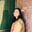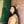Trusted answers to developer questions
Trusted Answers to Developer Questions

Related Tags

numpy
python

# What is numpy.prod() in Python?Umme Ammara

Grokking Modern System Design Interview for Engineers & Managers

Ace your System Design Interview and take your career to the next level. Learn to handle the design of applications like Netflix, Quora, Facebook, Uber, and many more in a 45-min interview. Learn the RESHADED framework for architecting web-scale applications by determining requirements, constraints, and assumptions before diving into a step-by-step design process.

Python’s numpy.prod() computes the product of the array elements over a specified axis.

## Syntax

numpy.prod() is declared as follows:

numpy.prod(a, axis=None, dtype=None, out=None, keepdims=<no value>, initial=<no value>, where=<no value>)


In the syntax above, a is the non-optional parameter, and the rest are optional parameters.

## Parameters

numpy.prod() takes the following non-optional parameter:

• a [array-like] - input array.

numpy.prod() takes the following optional parameters:

• axis [None, int, tuples of int] - axis along which we want the product computed. If the axis is None, numpy.prod() will compute the product across all the input array elements. If the axis is negative, the product is computed from the last to the first axis. An axis with a value of 0 will compute the product along the column, and an axis with a value of 1 will compute the product along the row.

• out [ndarray] - represents the location into which the output is stored.

• dytpe [dtype] - represents the returned array type and the accumulator in which the elements are summed. By default, the dtype of the input array is used.

• keepdims [boolean] - True value ensures that the reduced axes are left as dimensions with size one in the output. This ensures that the output is broadcasted correctly against the input array. If a non-default value is passed, keepdims will be passed through to the prod() method of sub-classes of ndarray. In the case of the default value, this will not be done.

• initial [scalar] - minimum value of the output element.

• where [array_like of bool] - represents the elements to compare for the minimum.

## Return value

numpy.prod() returns an array with the same shape as the input array, with the specified axis removed. If an output array is specified in the out parameter, a reference to out is returned.

## Examples

The following example outputs the product of the array arr where the axis parameter is not specified:

import numpy as nparr = np.array([1,2,5,6,9])print (np.prod(arr))

The example below outputs the product of the array arr1 where axis is specified as 0 and 1:

import numpy as nparr1 = np.array([[2,4,5], [7,10,1]])#Product along the columnsprint(np.prod(arr1, axis = 0))#Product along the rowsprint(np.prod(arr1, axis = 1))

RELATED TAGS

numpy
python

CONTRIBUTORUmme Ammara